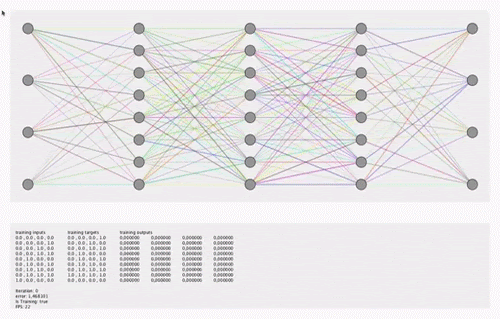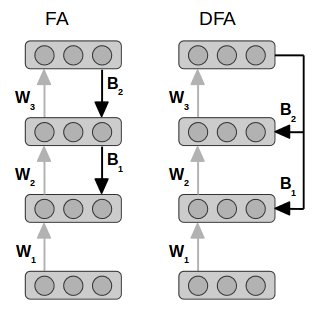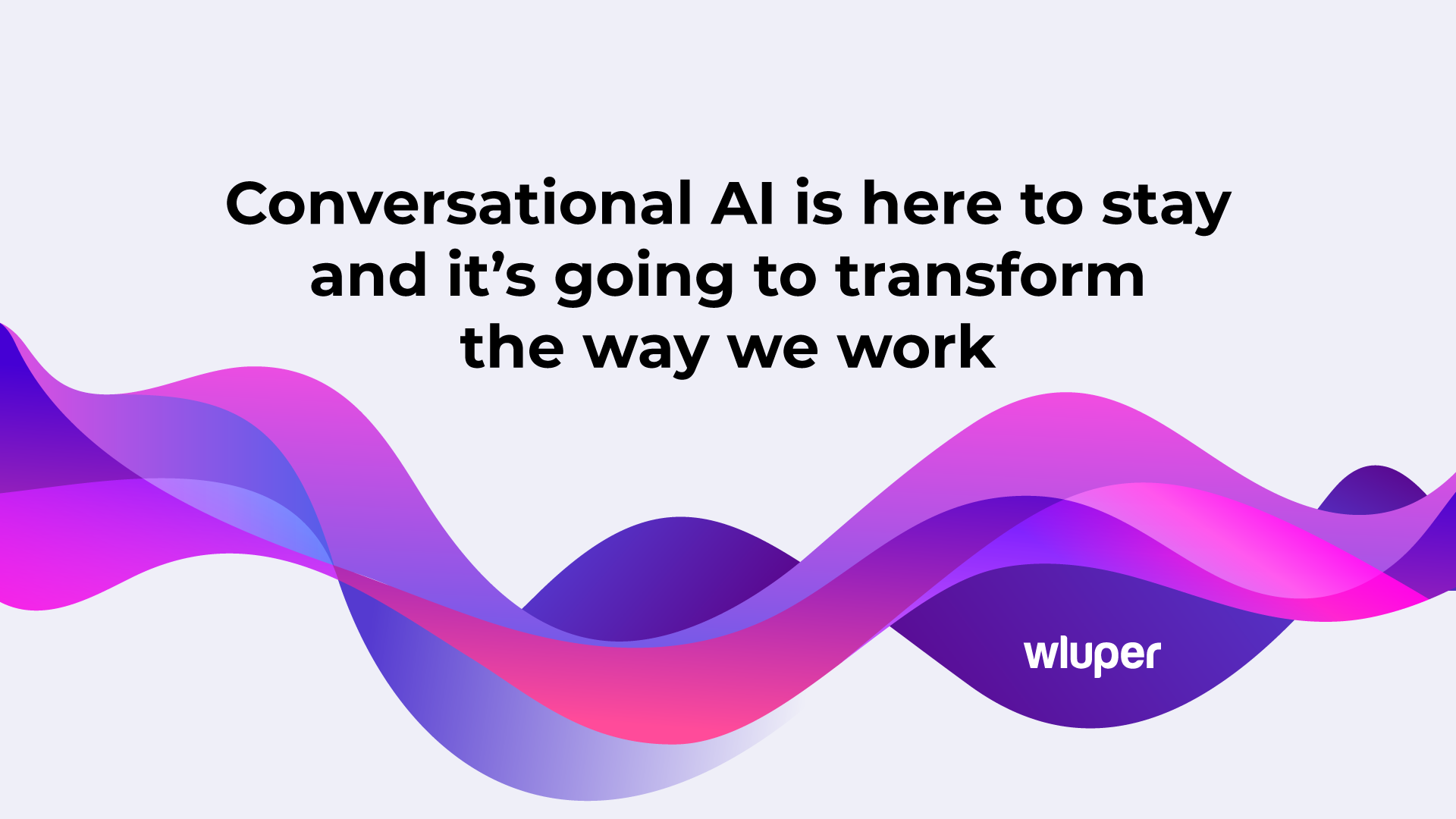# Deep-Learning 2.0? A Quicker, Cheaper Replacement to BackpropagationMohammed Terry-Jack

March 2, 2021

Neural Networks, especially the earlier, shallower ones (like those with a single hidden layer), have a range of learning algorithms, i.e. ways to train their weights. To name but a few:

• Gradient Descent (adjusting the weights incrementally, learning step by learning step, down the gradient/slope of error to minimise/reduce that error);
• Evolutionary techniques (to search for the optimal weights);
• Or perhaps one-shot learning methods (e.g. pseudo-inverse matrix methods used in Extreme Learning Machines);
• Or even simple, biologically-inspired algorithms like Hebbian learning (“neurons that fire together, wire together”) still used by some modern variants of neural networks (e.g. Hierarchical Temporal Memory, which, unlike most artificial neural networks, aims to model the human brain).

However, why aren’t many of these methods used for Deep Learning? Mainly because it is not easy to extend them to neural networks with deeper layers since many of the supervised learning signals which these methods depend upon are given only in respect to the final layer, and not for any of the deeper layers. For example, if a network has learnt to distinguish cars from horses, the final layer (which outputs the prediction of “car” or “horse”) can be checked against the supervised learning signal (the labels of each training example). However, the deeper layers which may learn to predict certain car features (e.g. wheels, windows and metallic textures), or features of horses (e.g. tail and legs) cannot be checked against the supervised signal since the training examples are not explicitly labelled with such features.

Back-propagation was revolutionary as it provided a way for learning algorithms, like Gradient Descent, to be extended to neural networks with multiple layers. A new age of Deep Learning was born.

By comparing network’s prediction to the supervision signal (i.e. the label “car”), we get a difference (the network’s error) which is also a gradient (slope) and thus the direction which the final weights can be adjusted to reduce that error (i.e. by descending the gradient). However, to apply gradient descent to the weights of deeper layers, we require the error gradients for each of those deeper layers. But, since we do not have any supervision signals corresponding to these layers (i.e. hierarchical feature labels of a car, like “wheels”, “windows”, etc) then we cannot use direct comparisons to obtain the error as we did with final layer.

Thanks to the back-propagation of errors, however, we are able to propagate a final layer’s error backward through each preceding layer, to approximate their own errors. Et voila! Problem solved.

Unfortunately, backprop is no cheap process. It heavily relies on costly calculations to compute things like the inverse of an activation function which also restricts the neural activation functions to those which are easier to differentiate, etc. Aren’t there any easier, cheaper ways to estimate the errors of prior layers? Well, at Wluper, we discovered one such simpler, cheaper alternative during this year’s NeurIPS conference. It is known as Feedback Alignment, or more precisely, Direct Feedback Alignment and Indirect Feedback Alignment (as well as other variants gaining popularity).

Feedback Alignment (FA) propagates the final layer’s error backward through each layer just like backprop. However, the weights in between each layer (through which the error propagates) are left completely random, static and untrained (as opposed to backprop which uses the Transverse of the incrementally fine-tuned weights also used in the forward pass during inference time). It seems that the network is still able to learn perfectly well when random weights are used to project the errors backward instead (this is because the forward weights also learn to align themselves in a way that make the random backward weights meaningful).Whereas Backprop (BP) propagates the error back through the incrementally-tuned weights, Feedback Alignment (FA) propagates it back through a separate set of static, untrained weights which remain completely random throughout training — and the network still learns!

Direct Feedback Alignment (DFA) is a newer variant which propagates the final layer’s error directly to the relevant prior layer bypassing any intermediate layers between the final layer and that particular layer (i.e. it passes the error to all layers in parallel)…

…instead of propagating the error back through each layer in-turn (i.e. sequentially) as with backprop and feedback alignment. This means that propagating the error backwards is not dependent on the errors of other prior layers — greatly simplifying the process of calculating prior errors!Feedback Alignment (FA) propagates the error back through layers sequentially, while Direct Feedback Alignment (DFA) directly passes errors to each layer in parallel.

Lets implement a simple Neural Network class to use with DFA:

<pre>class NeuralNetwork:
...
def infer(self, input):
layers,_= self.forward_pass(inputs)
return layers[-1]def forward_pass(self,inputs):
hidden_layers_activated,hidden_layers_logits = self._hidden_layers(inputs)
predicted_outputs = self._output_layer(hidden=hidden_layers_activated[-1])
hidden_layers_activated.append(predicted_outputs)
return hidden_layers_activated,hidden_layers_logitsdef _hidden_layers(self,layer):
activated_layers = []
unactivated_layers = []
for depth in range(self.N_HIDDEN_LAYERS):
logits = layer @ self.WEIGHTS[depth]
layer = self.activation_function(logits)
unactivated_layers.append(logits)
activated_layers.append(layer)
return activated_layers,unactivated_layersdef _output_layer(self, hidden):
return hidden @ self.WEIGHTS[-1]
</pre>

Now for the DFA related functions:

<pre>def direct_feedback_alignment(self, inputs, outputs, epochs,learning_rate):
desired_inputs = inputs
desired_outputs = outputs
for epoch in range(epochs):
error,loss = self.direct_feedback_alignment_step(desired_inputs,desired_outputs,learning_rate)def direct_feedback_alignment_step(self, desired_inputs, desired_outputs, learning_rate):
hidden_layers, unactivated_layers = self.forward_pass(desired_inputs)
predicted_outputs = hidden_layers.pop()
self.update_weights(
delta_weights=self.get_delta_weights_DFA(
error=predicted_outputs - desired_outputs,
hidden_layers= [desired_inputs] + hidden_layers,
random_weights=self.RANDOM_WEIGHTS,
unactivated_layers=unactivated_layers,
desired_inputs=desired_inputs,
n_layers=self.N_HIDDEN_LAYERS
),
learning_rate=learning_rate
)def update_weights(self, delta_weights,learning_rate):
for depth in range(self.N_HIDDEN_LAYERS):
self.WEIGHTS[depth+1] += learning_rate*delta_weights[depth]@staticmethod
def get_delta_weights_DFA(error,hidden_layers,random_weights,unactivated_layers,desired_inputs,n_layers):
delta_weights = []
e = da = error.T
for depth in range(n_layers,1,-1):
h = hidden_layers[depth-1]
B = random_weights[depth]
a = unactivated_layers[depth-1]
dW = da @ h
delta_weights.append(-dW.T)
da =  B @ e * self.differential_of_sigmoid(a).T
dW = da @ h
delta_weights.append(-dW.T)
return delta_weights[::-1]
</pre>

Indirect Feedback Alignment (IFA) is another variant which uses just one set of random weights (reducing the number of weights stored in memory) to project the final layer’s error back to the farthest hidden layer (bypassing any intermediate layers, like direct feedback alignment). Then, the error is propagated forward to each subsequent layer (via the weights being tuned like with backprop, except it is back-to-front — so…forwardprop). This also means there is no need to take the transverse of the weights as done when going in the reverse direction (as with backprop).

Wluper has built upon the concepts introduced by IFA to develop a simple, one-shot deep-learning variant. So stay tuned for more on that soon.

If you want to work on Conversational AI, check our careers page.

## Featured